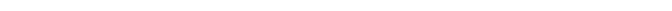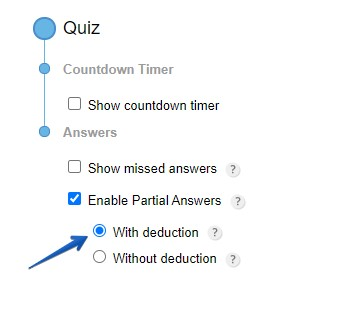# How do partial answer with deduction work? – CaptainForm WordPress PluginPartial answers with deduction cancel the score of a question if the number of incorrectly selected choices is equal to the correct ones. In other words, one incorrect answer given on the same question cancels the correct one.

Example: Question A has the available answers x,y,z, where y is the correct answer. If a user selects x and y, the correct answer will no longer be taken into account, because x canceled y. Each question is worth 100% and each available answer (or choice) is worth that 100% divided to the number of available choices for the question. In the example above, each choice is worth 33.33%.

Also, a wrong answer, if not selected, is considered a correct choice and contributes to the final score of the question. As choice z was not selected, it’s considered correct and receives 33.33%.

Quizzes that use partial answers with deduction are harder to beat, as form users would really need to know the answer to the question.You’ll find this feature in the SettingsAdvanced section of your form. Click the Enable Partial Answer checkbox and select With Deduction. Don’t forget to save your changes on top before leaving.